# Multiplication and Division, Part 2

Students deepen their understanding of multiplication and division, including their properties and extending their study of factors to include all units from 0 to 10, as well as multiples of 10 within 100.

Math

Unit 3

## Unit Summary

Unit 3 extends the study of factors from 2, 3, 4, 5, and 10, which students explored in Unit 2, to include all units from 0 to 10, as well as multiples of 10 within 100. To work with these more challenging units, students will rely on skip-counting (a Level 2 strategy) and converting to an easier problem (a Level 3 strategy dependent on the properties of operations). They then will apply their understanding of all four operations to two-step word problems as well as arithmetic patterns. Finally, the unit culminates with a focus on categorical data, where students draw and solve problems involving scaled picture graphs and scaled bar graphs, a nice application of the major work of multiplication and division.

Topic A begins by reminding students of the commutative property they learned in Unit 2, as well as introducing them to the distributive and associative properties, upon which they will rely for many of the strategies they learn for the larger factors. In order to be able to use these properties, they need to understand how to compute with a factor of 1, which they explore along with 0, as well as understand how to use parentheses. They’ll then explore the factors of 6, 7, 8, and 9 in Topics B and C. Because of the increased difficulty of these facts, students will rely on both skip-counting (a Level 2 strategy) as well as converting to an easier problem (a Level 3 strategy). Converting to an easier problem is dependent on the properties of operations (e.g., to find $$6\times7$$, think of $$5\times7$$ and add a group of 7 is dependent on the distributive property). Thus, students will work with the properties extensively throughout the unit, with their understanding of them and notation related to them growing more complex and abstract throughout the unit. In Topic D, students will multiply one-digit numbers by multiples of 10 and by two-digit numbers using the associative property. Then, students solve two-step word problems involving all four operations, assessing the reasonableness of their answer, and identify arithmetic patterns and explain them using the properties of operations. Finally, students explore picture graphs in which each picture represents more than one object and bar graphs where the scale on the axis is more than 1, a key development from Grade 2 (3.MD.3). As the Progressions note, “these developments connect with the emphasis on multiplication in this grade” (MD Progression, p. 7). Students also solve one- and two-step word problems related to the data in these plots, relying on the extensive work students have done with word problems throughout the year. Thus, this supporting cluster standard nicely enhances the major work they’ve been working on throughout this and the previous unit.

In Unit 3, students deepen their understanding of multiplication and division, including their properties. “Mathematically proficient students at the elementary grades use structures such as…the properties of operations…to solve problems” (MP.7) (Standards for Mathematical Practice: Commentary and Elaborations for K–5, p. 9). Students use the properties of operations to convert computations to an easier problem (a Level 3 strategy), as well as construct and critique the reasoning of others regarding the properties of operations (MP.3). Lastly, students model with mathematics with these new operations, solving one- and two-step equations using them (MP.4).

Students’ understanding of multiplication and division will further develop in Unit 4, when students study area. Students will also use their understanding of these operations in Unit 7 when they apply them in the context of measurement word problems. In subsequent years, students’ understanding will be entirely dependent on their conceptual understanding and fluency with these operations—everything from multi-step multiplicative comparison words problems in Grade 4 to polynomial multiplication and division in Algebra 2, and lots in between. Thus, this unit culminates major work of Grade 3 as well as deeply important foundational work upon which students will rely for years to come.

Pacing: 31 instructional days (28 lessons, 2 flex days, 1 assessment day)

For guidance on adjusting the pacing for the 2021-2022 school year, see our 3rd Grade Scope and Sequence Recommended Adjustments.

Fishtank Plus for Math

Unlock features to optimize your prep time, plan engaging lessons, and monitor student progress.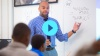## Assessment

This assessment accompanies Unit 3 and should be given on the suggested assessment day or after completing the unit.

## Unit Prep

### Intellectual Prep

#### Intellectual Prep for All Units

• Read and annotate “Unit Summary” and “Essential Understandings” portion of the unit plan.
• Do all the Target Tasks and annotate them with the “Unit Summary” and “Essential Understandings” in mind.
• Take the Post-Unit Assessment.

#### Unit-Specific Intellectual Prep

Read the following table that includes models used throughout the unit.

 Equal groups Example: 4 equal groups of 3 stars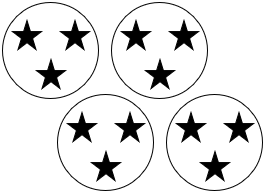Array Example: 4 rows of 3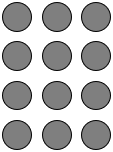Tape diagram Example: There are 4 bags with 3 plums in each bag. How many plums are there in all?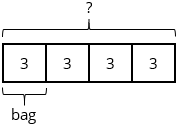Concrete or pictorial base ten blocks Example: Find $$2 \times 60$$ using base ten blocks.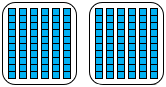Picture graph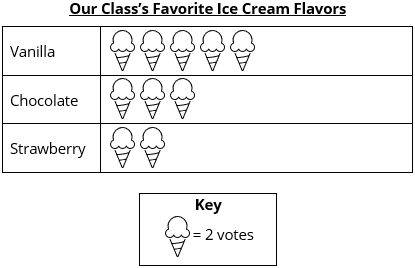Bar graph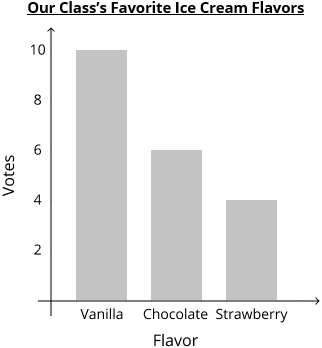### Essential Understandings

• Multiplication problems can be solved using a variety of strategies of increasing complexity, including making and counting all of the quantities involved in multiplication or division (Level 1 strategy), repeated counting on by a given number (Level 2), and using the properties of operations to compose and decompose unknown facts into known ones (Level 3).
• Unknown numbers in a count sequence can be found by using mental strategies. “For example, in the count-by for 7, students might use the following mental decompositions of 7 to compose up to and then go over the next decade, e.g., 14 + 7 = 14 + 6 + 1 = 20 + 1 = 21” (OA Progression, p. 25).
• Two, five, and ten are often helpful values to use when using properties to compose and decompose.
• Making sense of problems and persevering in solving them is an important practice when solving word problems. Key words do not always indicate the correct operation.

### Materials

• Base ten blocks (24 tens, 2 hundreds per student or small group) — Students might not need these depending on their reliance on concrete materials. Students can also use Paper base ten blocks cut into units instead. See Lesson 17 Anchor Task 1 Notes for more information.
• Multiplication Chart (1 per student)

### Vocabulary

associative property

distributive property

parentheses

key

scale

To see all the vocabulary for Unit 3, view our 3rd Grade Vocabulary Glossary.

## Unit Practice

Word Problems and Fluency Activities

Access daily word problem practice and our content-aligned fluency activities created to help students strengthen their application and fluency skills.## Lesson Map

Topic A: Introduction to The Properties of Operations

Topic B: Multiplication and Division by 6 and 7

Topic C: Multiplication and Division by 8 and 9

Topic D: Multiplication and Division by Values Greater than 10

Topic E: Two-Step Word Problems and Patterns in Arithmetic

Topic F: Scaled Picture and Bar Graphs

## Common Core Standards

Key

Major Cluster

Supporting Cluster

### Core Standards

#### Measurement and Data

• 3.MD.B.3 — Draw a scaled picture graph and a scaled bar graph to represent a data set with several categories. Solve one- and two-step "how many more" and "how many less" problems using information presented in scaled bar graphs. For example, draw a bar graph in which each square in the bar graph might represent 5 pets.

#### Number and Operations in Base Ten

• 3.NBT.A.3 — Multiply one-digit whole numbers by multiples of 10 in the range 10—90 (e.g., 9 × 80, 5 × 60) using strategies based on place value and properties of operations.

#### Operations and Algebraic Thinking

• 3.OA.A.3 — Use multiplication and division within 100 to solve word problems in situations involving equal groups, arrays, and measurement quantities, e.g., by using drawings and equations with a symbol for the unknown number to represent the problem.
• 3.OA.A.4 — Determine the unknown whole number in a multiplication or division equation relating three whole numbers. Determine the unknown whole number in a multiplication or division equation relating three whole numbers. For example, determine the unknown number that makes the equation true in each of the equations 8 × ? = 48, 5 = _ ÷ 3, 6 × 6 = ?
• 3.OA.B.5 — Apply properties of operations as strategies to multiply and divide. Students need not use formal terms for these properties. Example: Knowing that 8 × 5 = 40 and 8 × 2 = 16, one can find 8 × 7 as 8 × (5 + 2) = (8 × 5) + (8 × 2) = 40 + 16 = 56. (Distributive property.) Example: If 6 × 4 = 24 is known, then 4 × 6 = 24 is also known (Commutative property of multiplication.) 3 × 5 × 2 can be found by 3 × 5 = 15, then 15 × 2 = 30, or by 5 × 2 = 10, then 3 × 10 = 30. (Associative property of multiplication.)
• 3.OA.C.7 — Fluently multiply and divide within 100, using strategies such as the relationship between multiplication and division (e.g., knowing that 8 × 5 = 40, one knows 40 ÷ 5 = 8) or properties of operations. By the end of Grade 3, know from memory all products of two one-digit numbers.
• 3.OA.D.8 — Solve two-step word problems using the four operations. Represent these problems using equations with a letter standing for the unknown quantity. Assess the reasonableness of answers using mental computation and estimation strategies including rounding. This standard is limited to problems posed with whole numbers and having whole-number answers; students should know how to perform operations in the conventional order when there are no parentheses to specify a particular order (Order of Operations).
• 3.OA.D.9 — Identify arithmetic patterns (including patterns in the addition table or multiplication table), and explain them using properties of operations. For example, observe that 4 times a number is always even, and explain why 4 times a number can be decomposed into two equal addends.

• 2.MD.D.10

• 2.NBT.A.1
• 2.NBT.A.2
• 3.NBT.A.2

• 2.OA.A.1
• 2.OA.C.3
• 2.OA.C.4
• 3.OA.A.1
• 3.OA.A.2
• 3.OA.B.6

• 4.NBT.B.5
• 4.NBT.B.6

• 4.NF.B.4
• 5.NF.B.3
• 5.NF.B.4
• 5.NF.B.5
• 5.NF.B.6
• 5.NF.B.7

• 4.OA.A.1
• 4.OA.A.2
• 4.OA.A.3
• 4.OA.B.4

### Standards for Mathematical Practice

• CCSS.MATH.PRACTICE.MP1 — Make sense of problems and persevere in solving them.

• CCSS.MATH.PRACTICE.MP2 — Reason abstractly and quantitatively.

• CCSS.MATH.PRACTICE.MP3 — Construct viable arguments and critique the reasoning of others.

• CCSS.MATH.PRACTICE.MP4 — Model with mathematics.

• CCSS.MATH.PRACTICE.MP5 — Use appropriate tools strategically.

• CCSS.MATH.PRACTICE.MP6 — Attend to precision.

• CCSS.MATH.PRACTICE.MP7 — Look for and make use of structure.

• CCSS.MATH.PRACTICE.MP8 — Look for and express regularity in repeated reasoning.

Unit 2

Multiplication and Division, Part 1

Unit 4

Area